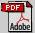Applied Rheology: PublicationsSamer Alokaily, Kathleen Feigl, Franz X. Tanner, Erich J. Windhab
Numerical Simulations of the Transport of Newtonian and Non-Newtonian Fluids via Peristaltic Motion

Appl. Rheol. 28:3 (2018) 32832 (15 pages)

Abstract: Two geometrical models are developed to simulate fluid transport via peristaltic motion in tubes of uniform or linearly decreasing radius: A 2-D axisymmetric tubular model and a 2-D axisymmetric conical model. In both models, peristaltic motion is induced by a traveling wave along the wall of the computational domain which deforms the wall and the computational mesh. These geometrical models are coupled with a finite volume solver from the open source software package OpenFOAM which is used to simulate the peristaltic flow for different Newtonian and non-Newtonian fluids in the laboratory (or Eulerian) frame of reference. After validation of the solver with experimental data, simulations are performed in each geometrical model to determine the influence of a given set of parameters on peristaltic flow behavior and transport efficiency. The parameters that are varied include the wave speed, relative occlusion, Newtonian viscosity, and power-law index for shear-thinning non- Newtonian fluids. For both computational models, the transport efficiency is found to increase strongly with relative occlusion, to decrease as the amount of shear-thinning increases, and to be independent of wave speed. In the tubular model, transport efficiency is found to be independent of Newtonian viscosity, while in the conical model, it decreases as viscosity decreases for Reynolds numbers greater than one. © 2018 Applied Rheology.

-- full text PDFavailable for subscribers --

-- open access PDF extractavailable for non-subscribers --